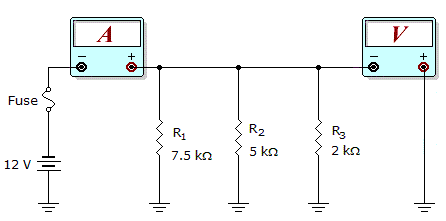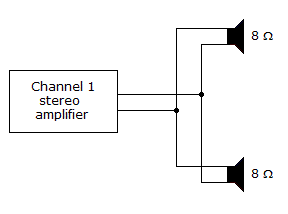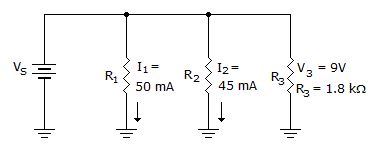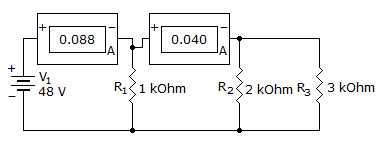# Electronics - Parallel Circuits

### Exercise :: Parallel Circuits - General Questions

11.What would these meter readings indicate about the circuit in the given circuit?

Meter Readings: I = 7.6 mA, V = 12 V

 A. R1 is open. B. R2 is open. C. The fuse is open. D. The circuit is operating normally.

Explanation:

No answer description available for this question. Let us discuss.

12.In the given circuit, Channel 1 of the stereo amplifier outputs 12 V to the speakers. How much total power is the amplifier delivering to the speakers?

 A. 0 W B. 18 W C. 36 W D. More information is needed to find the total power delivered to the speakers.

Explanation:

No answer description available for this question. Let us discuss.

13.

If a 1 kand a 2 kresistor are parallel-connected across a 12 V supply, how much current is received by the 2 kresistor?

 A. 4 mA B. 6 mA C. 8 mA D. 12 mA

Explanation:

No answer description available for this question. Let us discuss.

14.What does VS equal in the given circuit?

 A. 0 V B. 9 V C. 27 V D. More information is needed to find VS.

Explanation:

No answer description available for this question. Let us discuss.

15.

The current through R1 will be:A. 128 mA B. 88 mA C. 48 mA D. 2.2 mA